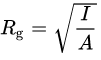# Radius Of Gyration | Definition | Formula |Radius of gyration of a body is nothing but the radial distance of that point, from the axis of rotation at which the whole mass of the body is supposed to be concentrated. It is always calculate about an axis of rotation. Radius of gyration is also called as gyradius and denoted by “ R“.

Let’s Consider a body of mass ‘M’ which is rotating about an axis which is passing through point ‘O’ and perpendi2cular to the horizontal plane of axis of axis of rotation. So According to law, About this axis of rotation the moment of inertiaI” of the body is given below.

In this figure above the mass of the body is ‘M’. The radial distance from the axis of rotation is k. So its moment of inertia remains the same about that axis of rotation then, ‘K’ is called the radius of gyration of the given body having mass M. Therefore the moment of inertia and radius of gyration of the body is given below in term of formula.

### What is radius of gyration formula

I=MR2 therefore :## What is radius of gyration in simple words

The radius of gyration for a given body, mass always remains constant. On changing the axis of rotation, the distance of particles also gets change. Therefore radius of gyration (K) is also gets change. The radius of gyration is always depends upon the shape and size of the given body. The main significance is that It measures the distribution of mass around the axis of rotation. Less value of radius of gyration shows that the mass of the body is distributed near to the axis of rotation and large value of radius of gyration, shows the distribution of mass of the body is at large distance from the axis of rotation.

### What is the SI unit of radius of gyration

In c.g.s system, radius of gyration is expressed in cm. In SI system, radius of gyration is expressed in m.

### What is the Dimensions of radius of gyration

The dimensions of radius of gyration is [R] = [L1 M0 T0] .